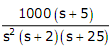# The first two rows of Routh's tabulation of a third order equation are as follows. This means there are

1.  two roots at s = ±j and one root in right half s-plane

2.  two roots at s = ±j2 and one root in left half s-plane

3.  two roots at s = ±j2 and one root in right half s-plane

4.   two roots at s = ±j and one root in left half s-plane

4

two roots at s = ±j and one root in left half s-plane

Explanation :
No Explanation available for this question

# The asymptotic approximation of the log-magnitude vs frequency plot of a system containing only real poles and zeros is shown.Its transfer function is

1.

2.

3.

4.

4Explanation :
No Explanation available for this question

# The trace and determinant of a 2x2 matrix are known to be -2 and -35 respectively.Its eigen values are

1.  -30 and -5

2.  -37 and -1

3.  -7 and 5

4.  17.5 and -2

4

-7 and 5

Explanation :
No Explanation available for this question

# The following circuit has R=10kΩ,C=10F μR.The input voltage is a sinusoid at 50Hz with an rms value of 10V. Under ideal conditions, the current is from the source is

1.  10n mA leading by 900

2.  20n mA leading by 900

3.  10 mA leading by 900

4.  10n mA lagging by 900

4

10n mA lagging by 900

Explanation :
No Explanation available for this question

# In the figure shown, all elements used are ideal. For time t

1.  V

2.  2V

3.  1.5V

4.  3V

4

1.5V

Explanation :
No Explanation available for this question

# Transformer and emitter follower can both be used for impedance matching at the output of an audio amplifier. The basic relationship between the input power Pin and output power Pout in both the cases is

1.  Pin = Pout for both transformer and emitter follower

2.  Pin > Pout for both transformer and emitter follower

3.  Pin < Pout for transformer and Pin = Pout for emitter follower

4.  Pin = Pout for transformer and Pin < Pout for emitter follower

4

Pin = Pout for both transformer and emitter follower

Explanation :
No Explanation available for this question

# The equivalent capacitance of the input loop of the circuit shown is

1.  2hμF

2.  100hμF

3.  200hμF

4.  4hμF

4

2hμF

Explanation :
No Explanation available for this question

# In an 8085 microprocessor,the contents of the Accumulator,after the following XRA A.instructions are executed will become MVIB F0H

1.  01 H

2.  0F H

3.  0F H

4.  10 H

4

10 H

Explanation :
No Explanation available for this question

# For the Y-bus matrix of a 4-bus system given in per unit,the buses having shunt elements are

1.  3 and 4

2.  2 and 3

3.  1 and 2

4.  1,2 and 4

4

1 and 2

Explanation :
No Explanation available for this question

1.  0.5

2.  2

3.  4

4.  6

4